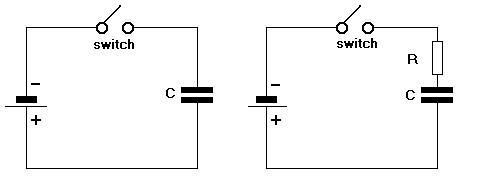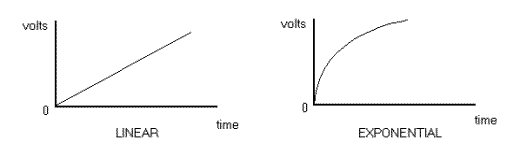Home > Electronic Tutorials > General Theory > Time Constants Tutorial

General Theory - Electronic Tutorials

# Time Constants Tutorial

Like charges repel, unlike attract.  In the first diagram, when the switch is closed, the negative terminal of the battery repels the negative electrons and pushes them onto the upper plate of the capacitor C.Similarly, the positive terminal attracts the negative electrons away from the lower plate. If the battery is now removed, C remains charged up to the battery voltage. This can be dangerous, since capacitors can remain charged to high voltages for a long time. If a screwdriver is now placed across the capacitor terminals, the surplus electrons on the upper plate will now flow to the lower plate.

The C is now discharged.

Doing this can also be dangerous.

The screwdriver has a low resistance, and Mr Ohm says "low resistance means high current". One vapourised screwdriver !!

Therefore large, highly charged capacitors must be discharged via a resistor, to limit the amount of discharge current that can flow.

In the second diagram, a resistor R has been placed in series with C. When the switch is closed, C charges from the battery, as described previously. The charging current passes through R. Since R limits the amount of current that can flow (Ohms law), C takes time to charge up to the battery voltage.

The larger the values of C and R, the longer C takes to charge. Liken it to filling a bucket with a hosepipe. The larger the bucket (C), and the more you stand on the hosepipe (R), then the longer it takes to fill the bucket. The value of C in Farads, multiplied by the value of R in ohms, gives us the TIME CONSTANT (RC), measured in seconds.

If C = 2 Farads and R = 10 ohms then RC = 20 seconds. This means that C will take 20 seconds to charge up to 63 % of the battery voltage. If it is a 100 volt battery, then after 20 seconds, the capacitor voltage will be 63 volts.

If we draw a graph of the increase of capacitor voltage against time, then we get a curve that is not linear ( not a straight line).

The curve is exponential. It increases rapidly at the start and then slows down. It gets slower and slower.If C is discharged, by connecting a resistor across it, then the capacitor voltage falls BY 63 % after RC seconds.

Time constants are often used where a time delay is required.

Note: To report broken links or to submit your projects please send email to Webmaster

 Discover